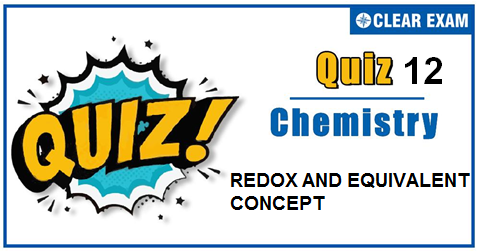## [LATEST]\$type=sticky\$show=home\$rm=0\$va=0\$count=4\$va=0

The one subject in NEET which is candidates who can easily attain good marks is Chemistry. That's the reason, often, one doesn't pay notice and choose to compromise it. But if one wants to rank above others, the tip is to be thorough with NEET chemistry concepts. The understanding of reactions and definite basic understanding is what requires major attention in Chemistry but once done it only gets simpler from there. The main focus on the to-do list should be on getting a hang of the NCERT syllabus of NEET chemistry. .

Q1. A chemical balance used normally for weighing in laboratory can weigh upto a least count of :
•  0.0001 g
•  0.001 g
•  0.0002 g
•  0.002 g
Solution
The weight of rider used is 0.0002 g.

Q2.The oxidation state of Fe in Fe3O4 is
•  +3
•  8/3
•  +6
•  +2
Solution
Let the oxidation state of Fe in Fe_3 O_4 = x ∴3x+4×(-2)=0 Or 3x-8=0 ∴ x=8/3

Q3.  In the preparation of chlorine from HCl,MnO2 acts as :
•  Reducing agent
•  oxidising agent
•  Catalytic agent
•  Dehydrating agent
Solution
Mn(4+)+2e ⟶Mn(2+); MnO2 is itself reduced.

Q4. The eq. wt. of Na2 S2 O3 as reductant, in the reaction, Na2S2O3+5H2 O+4Cl2 ⟶2NaHSO4+8HCl∶
•  (Mol.wt.)/1
•  (Mol. wt.)/2
•  (Mol. wt.)/6
•  (Mol. wt.)/8
Solution
S2(2+) ⟶2S(6+)+8e

Q5.Which one is not a redox titration?
•  FeSO4 vs.K2 Cr2O7
•  CuSO4 vs.hypo
•  I2 vs.hypo
•  AgNO3 vs.KCl
Solution
It is precipitation reaction.

Q6. What volume of 3 molar HNO3 is needed to oxidise 8 g of Fe(2+) to Fe(3+)? HNO3, gets converted to NO :
•  8 mL
•  16 mL
• 32 mL
•  64 mL
Solution
Meq.of HNO3=Meq.of Fe(2+) (Eq.wt.of HNO_3=M/3) Or 3 × 3 × V=8/56 × 1000 ∴V=15.87 mL

Q7.In the equation , CrO42+SO32 Cr(OH)4+SO42 the oxidation number of Cr changes from
•  6 to 4
•  6 to 3
•  8 to 4
•  4 to 3
Solution
CrO4(2-)+SO3(2-)⟶Cr(OH)4-+SO4(2-) Let the oxidation number of Cr is x in CrO4(2-) x+4(-2)= -2 x= 6 and in Cr(OH)4- the oxidation number of Cr is y y+4(-2)+4(1)= -1 y-8+4=-1 y=3 Hence, oxidation number of Cr changes from +6 to +3.

Q8.Maximum oxidation state is present in :
•  CrO2 Cl2 and MnO4-
•  TMnO2
•  [Fe(CN)6](3-) and [Co(CN)6 ](3-)
•  MnO
Solution
Cr in CrO2 Cl2 has +6 and Mn in MNO4- has +7 oxidation number respectively, the highest value for them.

Q9.The equivalent weight of iron in Fe2 O3 would be :
•  18.6
•  28
•  56
•  11
Solution
TFe2 O3 ∴ Total charge on cation or anion = +6 Fe2(3+) O3(2-) ∴ E=112/6 or 56/3

Q10. Which acts as reducing agent as well as oxidising agent?
•  O3
•  ClO4-
•  F2
• MnO4-
Solution
F2 is oxidant; ClO4- and MnO4- are also oxidant.## Want to know more

Please fill in the details below:

## Latest NEET Articles\$type=three\$c=3\$author=hide\$comment=hide\$rm=hide\$date=hide\$snippet=hide

Name

ltr
item
BEST NEET COACHING CENTER | BEST IIT JEE COACHING INSTITUTE | BEST NEET & IIT JEE COACHING: REDOX AND EQUIVALENT CONCEPT QUIZ-12
REDOX AND EQUIVALENT CONCEPT QUIZ-12